Entropy Based Multi-criteria Decision Making Method under Fuzzy Environment and Unknown Attribute Weights | OMICS International
Global Journal of Technology and Optimization

# Entropy Based Multi-criteria Decision Making Method under Fuzzy Environment and Unknown Attribute Weights

Harish Garg1*, Nikunj Agarwal2 and Alka Choubey2

1School of Mathematics and Computer Applications, Thapar University, Patiala 147004, India

2Department of Mathematics, Jaypee Institute of Information and Technology, Noida-201307, U.P, India

Corresponding Author:
Harish Garg
School of Mathematics and
Computer Applications
Thapar University Patiala
147004, India
Tel: +91-86990-3114
E-mail: [email protected]

Received Date: March 11, 2015 Accepted Date: April 08, 2015 Published Date: April 30, 2015

Citation: Garg H, Agarwal N, Choubey A (2015) Entropy Based Multi-criteria Decision Making Method under Fuzzy Environment and Unknown Attribute Weights. Global J Technol Optim 6:182. doi:10.4172/2229-8711.1000182

Copyright: © 2015 Garg H, et al. This is an open-access article distributed under the terms of the Creative Commons Attribution License, which permits unrestricted use, distribution, and reproduction in any medium, provided the original author and source are credited.

Visit for more related articles at Global Journal of Technology and Optimization

#### Abstract

The main purpose of this paper is to investigate the multi-criteria decision making (MCDM) under the completely unknown attributes weights. As the information collected from the various resources related to different criteria for assessing the best alternatives is always imprecise in nature. Thus, to handle the impreciseness in the data, fuzzy set theory has been used during the analysis and representation each attribute in the form of triangular fuzzy numbers. Moreover, the attribute weight vectors, used for aggregating the decision maker’s preferences, are found by using an entropy function. Finally, a house selection example, has been taken for demonstrating the approach.

#### Keywords

Multi-criteria decision making; Entropy functions; Attribute weights; Linguistic variables

#### Introduction

Decision making is one of the most significant and omnipresent human activities in business, service, manufacturing, selection of products, etc. As it is quite common that the different attributes play a significant role during the selection of the best alternatives among the existing ones. During their selection, one of the significant issues is to find the proper standardized weight vectors which are useful for aggregating the different decision maker’s preferences. Technique for order preference by similarity to an ideal solution (TOPSIS), known as a classical multiple attribute decision making (MADM) method, has been developed by Hwang and Yoon  for solving the MADM problem. It is based on the idea that the chosen alternative should have the shortest distance from the positive ideal solution, and, on the other side, the farthest distance from the negative ideal solution. Traditionally, it has been generally assumed that all the information which access the alternative in terms of criteria and their corresponding weights are expressed in the form of crisp numbers . In spite of its popularity, the method is often criticized for its inability to adequately handle the inherent uncertainty and imprecision associated with the mapping of a decision-maker’s perception to crisp numbers.

Moreover, from the survey, it has been observed that the final ranking order of alternatives highly depends on the attribute weights and hence the proper assessment of the attributes weights play a dominant role in the decision-making process. Based on the information acquisition, the attribute weight in multi-criteria decision making is classified as subjective as well as objective. The former ones are determined by preference information on the attributes as given by the decision-maker while the latter one are determined by the decisionmaking matrix. The Shannon entropy method [1,21] is one of the most famous approach for determining the objective attribute weights, which express the relative intensities of attribute importance to signify the average intrinsic information transmitted to the decision maker. Thus, it is an important task for finding the proper attribute weights which will help the decision maker for obtaining the efficient decision under a reasonable time.

Therefore, this paper continues to study the multi-criteria decision making problems under the fuzzy environment with complete unknown attributes weight information. In the present paper, we extend the approach of TOPSIS to develop a methodology for solving the MADM problem in fuzzy environment. Considering the fuzziness in the decision data, linguistic variables are used to rate the each alternative with respect to each criterion and then convert the decision matrix into the fuzzy decision matrix. The unknown attributes weights of each criteria have been given by using the fuzzy entropy measurement and assessment of the different preferences for rating the attributes are represented in the form of linguistic variables in terms of triangular fuzzy numbers instead of crisp numbers. Based on these attributes weight and fuzzy decision matrix we construct a weighted normalized fuzzy decision matrix once the decision makers’ fuzzy ratings have been pooled. The lower bound value of alternatives has been designed to obtain the distance value of the corresponding alternatives for detecting the fuzzy positive ideal solution (FPIS) and the fuzzy negative ideal solution (FNIS). Finally, a closeness coefficient is defined for each alternative, to determine the rankings of all alternatives. The higher value of closeness coefficient indicates that an alternative is closer to FPIS and farther from FNIS simultaneously.

The rest of the manuscript is summarized as follows. Section 2 discusses the basic primilaries related to fuzzy set theory. Section 3describes the entropy based method for MCDM. A numerical example is utilized to illustrate the MCDM applicability of the proposed method in section 4. Finally, some concrete conclusion drawn is presented in Section 5.

Basic primilaries related to fuzzy set theory

Fuzzy set theory: Fuzzy set  is treated as a generalization of the classical set theory where the membership functions are only treated by their belonginess or not. In fuzzy set theory, this relaxation is overlapped with the condition that it allows the partial membership between 0 and 1 also. Based on it, the fuzzy set AÍ�i in the universe of discourse U is defined as the set of ordered pairs AÍ�i ={< x, μAÍ� (x)>| xÏµU}; where μAÍ� (x) represents the membership function of x in the fuzzy set AÍ�i such that 0 ≤ μAÍ�(x) ≤ 1.

Convex and normal set

A fuzzy set is said to be convex fuzzy set if the following inequality holds for two points x1,x2 ÏµU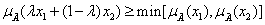and if there is atleast one element x ÏµU such that μAÍ�(x) =1 then that set is called as normal set.

Fuzzy numbers

A triangular fuzzy number AÍ�=<am1,a2,a3> such that a1 ≤ a2 ≤ a3 and all a1 ≤ a2 ≤ a3 Ïµ R is a convex and normal fuzzy set of the real line R such that μAÍ�(x) is a piecewise continuous and there exist at least one xo ÏµU such that μAÍ� (xo) =1 and their corresponding membership function is given by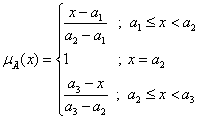Here, a1 represents the smallest possible bound, a2 represents the modal (crisp) value and a3represents the largest possible value that describes the fuzzy events.

Distance between the fuzzy triangular numbers

Let aÍ�=(a1,a2,a3) and bÍ�=(b1,b2,b3) be two triangular fuzzy numbers, then the distance between them is computed as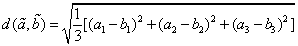Linguistic variables

The fuzzy theory provides the mechanism for representing the linguistic constructs such as “many”, “low”, “medium”, “few”, etc. In general, fuzzy logic provides an inference structure that enables appropriate human reasoning capabilities. A linguistic variable is characteristic by (X,T,U,M) where X is the name of the variable, T is the set of the terms of X, Uis the universe of discourse and M is the semantic rule for associating each term with a fuzzy set in U Here, in this paper, triangular fuzzy numbers have been used for representing the linguistic variable in the scale of 0 to 1 for rating the criteria and the alternative. These various linguistic variables are represented as very poor (VP), poor (P), medium poor (MP), fair (F), medium good (MG), good (G), and very good (VG).

#### Entropy Based Multi-criteria Decision making Approach

The multi-criteria decision making (MCDM) is a very practical method in the real world, and have very significant effect on both theory and practical. The aim of the MCDM is to find the best alternative among the given criteria. In this section, we shall investigate the multiple criteria decision making problems under fuzzy environment and the attribute weight to each criteria is computed based on defining their corresponding entropy method. For a MCDM problem, assume that a set of option/alternatives A1, A2,…Am to be considered under the set of n evaluation criteria C1,C2,…Cm. Assume that wj be the weight of criteria Cj where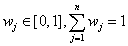Let there be

a prioritization between the attributes expressed by the linear ordering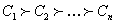(indicating that the attribute C1 has a higher priority than Cj, if i<j). Let Gij is the performance rating of the alternative Ai (i=1,2,…m). under criteria Cj(j=1,2,…,n) which are expressed in the form of fuzzy decision matrix and aij=(aij, bij, cij) be an attribute value provided by the decision maker for the alternative Ai with respect to the attribute Cj, where aij represents the lower value, bij represents the modal value and cij represents the upper value of the attribute and then the corresponding fuzzy decision matrix is expressed as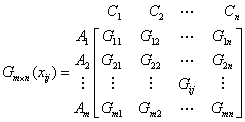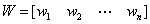Then we have the following decision making method which consists of the following steps for evaluation.

Step 1: Assignments of rating to the criteria and the alternatives: - The performance rating of each decision maker Dk (k=1,2,…K) for each alternative Aj (j=1,2,…m) with respect to criteria Cj (j=1,2,…n) are assessed in the form of triangular fuzzy numbers and their corresponding matrix is denoted by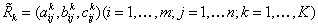. Based on these fuzzy rating, the aggregating fuzzy rating xÍ�ij of alternatives with respect to each criteria are given by xÍ�ij=(aij, bij, cij) where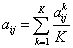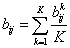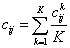Step 2: Normalize the fuzzy decision matrix: Normalize each attribute value xij (i=1,2…m; j=1,2…n) in the matrix RÍ�ik into the corresponding element in the matrix called normalized fuzzy decision matrix RÍ�i=[rÍ�iij]m×n, where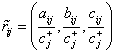and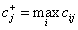(benefit criteria);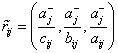and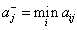(cost criteria).

Step 3: Determination of the entropy matrix: Based on the constructed normalized matrix RÍ�i in step 2, the weights corresponding to each attribute wj (j=1,2,...n) can be calculated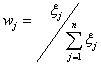where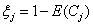is called the degree of divergence and E (Cj) is the information entropy  of attribute Cj which is calculated as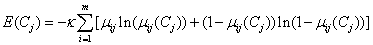where, κ is constant such that 0 ≤ E (Cj) ≤ 1.

Step 4: Compute the fuzzy PIS and fuzzy NIS: The fuzzy PIS and fuzzy NIS of the alternatives are calculated as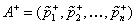where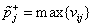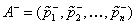where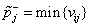where

vÍ�iij=rÍ�iij . wÍ�ij is the normalized weighted matrix VÍ�i=[vÍ�iij]m×n.

Step 5: Compute distance from each alternative: Let dij and d+ij be distanced from alternatives to the ideal solution (or negative ideal solution) i.e. distance of vij to pij and p+j respectively, therefore,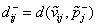and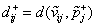Let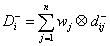and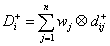for i = 1,2,..,m

be representing their corresponding weighted distance. Thus, a weighted distance of i d can be expressed by[ D-i D+i ] . Let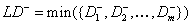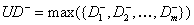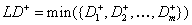and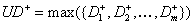be the fuzzy minimum and maximum values D-i,D+i Therefore,d-i denote the distance from [D-i,D+i] to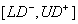and d+i denote the distance from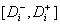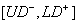Thus,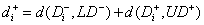and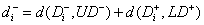for i =1,2......,m , where d(aÍ�i,bÍ�i) is the distance measurement between two fuzzy numbers aÍ�i and bÍ�i.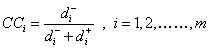Step 7: Rank the alternatives. Finally, rank all the alternatives Ai according to the closeness coefficient in decreasing order..

#### Illustrative Example

In order to demonstrate the applications of the develop methodology, we will consider an example where consists of 3 houses H1, H2, H3 examined by the four experts under fuzzy environment for the selection of houses against the 4 criteria or parameters C1, C2, C3, C4 defined as;

C1 :expensive,

C2 : Beautiful,

C3 :In good repair,

C4 :In good surrounding.

In order to take the decision related to these houses, four experts give the linguistic performance ratings, VP, P, MP, F, MG, G, and VG, in terms of fuzzy numbers which are as follows:

Very Poor (VP)=(0,0,0.2),

Poor (P)=(0,0.2, 0.4),

Most Poor (MP)=(0.2, 0.4, 0.5),

Fair (F)=(0.4, 0.5, 0.6),

Most Good (MG)=(0.5, 0.6, 0.8),

Good (G)=(0.6, 0.8, 1.0),

Very Good (VG)=(0.8, 1, 1)

of the three houses. These linguistic performance ratings are shown in Table 1. Thus, by using step 1 of the proposed approach, the average rating of the three houses by the experts in terms of fuzzy numbers are summarized in Table 2.

Gij H1 H2 H3
C1 {MG, G, G, VG} {VG, G, MG, MG} {MG, F, MG, F}
C2 {MG, VG, G, MG} {G, G, VG, G} {G, VG, G, G}
C3 {F,F,F, MG} {VG, G, MG, G} {VG, VG, G, G}
C4 {VG, G, VG, VG} {F, MG, MG, MG} {MG, MG, G, MG}

Table 1: Linguistic performance rating of the three houses.

Gij H1 H2 H3
C1 (0.625, 0.80, 0.95) (0.60, 0.75, 0.90) (0.45, 0.55, 0.70)
C2 (0.600, 0.75, 0.90) (0.65, 0.85, 1.00) (0.65, 0.85, 1.00)
C3 (0.425, 0.525, 0.65) (0.625, 0.80, 0.95) (0.70, 0.90, 1.00)
C4 (0.75, 0.95, 1.00) (0.475, 0.575, 0.75) (0.525, 0.65, 0.85)

Table 2: Average rating of three houses on four criteria.

Based on the entropy function given in Equation (1), the attribute weights are computed as w1=0.2665; w2=0.2578; w3=0.2185; w4=0.2570 and hence FPIS of each alternative are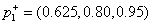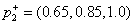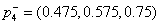while FNIS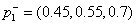are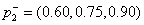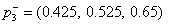. By using this information, the minimum and maximum bounds are UD+=0.1206, LD+=0.06957, UD-=0.1390 and LD+=0.1157. Hence, the distance of each alternative from its FPIS and FNIS are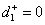,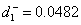,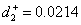,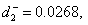,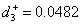and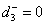Thus, the evaluated results about the houses are computed by using the closeness coefficient Thus, the evaluated results about the houses are computed by using the closeness coefficient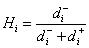as H1=1, H2=0.5560, H3=0. Therefore, ranking of the houses are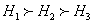and hence H1 is the most desirable one while H3 is the least one. Thus, H1 will be the best house to be purchased.

#### Discussion

As the attribute weights are the most important parameter for the decision maker for accessing the best alternative. So, in order to compare the proposed results with the existing techniques [6,12,17], the corresponding approaches have been implemented here for the considered data with the attribute weights w1=0.2665; w2=0.2578; w3=0.2185; w4=0.2570 and found that the proposed results coincides with the ones shown one shown in [6,12,17]. Thus, it has been concluded from the aforementioned results that the proposed decision making method can be suitably utilized to solve the multiple and decision making problem with completely unknown attribute weights.

#### Conclusion

In the present work, an approach for selecting the best alternative under the different selection or criteria is implemented. The approach has been illustrated through a case study of selected the house against the different criteria. The preferences of the various alternatives are represented in terms of linguistic variation as a triangular fuzzy number. As the key issues of decision making with incomplete weight information are to find the proper way to derive the weight vector and to aggregate the decision makers’ preferences. For this, entropy based method has been proposed for finding the attribute weights corresponding to each objective. Based on their weights, all the individual decision makers’ opinion for rating the candidate are aggregated using the fuzzy positive and negative ideal solutions. A ranking of the different alternatives has been done by using the closeness coefficient and based on that the best alternative is selected. The proposed method provides us with a useful way for dealing with multi-criteria fuzzy decision making problems within attribute weights. Although, we have focused on the MCDM in fuzzy environment in this paper, we have extended the present work in the direction of taking intuitionistic fuzzy numbers and intervalvalued intuitionistic fuzzy numbers. The work can also be extended to devise a suitable optimization model for deriving the attribute weights corresponding to alternative and hence best alternative is chosen according to them. Finally, different operators may use to aggregate the different alternatives and hence based on that ranking of the different alternative done.

#### References

Select your language of interest to view the total content in your interested language

### Article Usage

• Total views: 14229
• [From(publication date):
December-2015 - Dec 16, 2019]
• Breakdown by view type
• HTML page views : 9953

## Review summary

1. manoj
Posted on Aug 20 2016 at 4:22 pm
Wanted to read this paper being practically useful.Can't read the image? click here to refresh